TitleCollege Algebra
Tutorial 32B:
Inverse FunctionsLearning Objectives

 After completing this tutorial, you should be able to: Verify inverse function. Find the inverse of a function. Graph a function and its inverse on the same rectangular coordinate system. Determine that a function has an inverse by using the horizontal line test.Introduction

 In this tutorial we are going to learn about inverse functions.  Inverse functions are functions in their own right …they take on all of the same attributes. If you need a review on functions, feel free to go to College Algebra Tutorial 30: Introduction to Functions. What is special about the inverse function is its relationship to the function to which it is an inverse.  We will learn how to determine if a function has an inverse and if so how to find it.  Basically what happens is that x and y get interchanged and you solve for y, which forces you to apply inverse operations.  Consequently, you will find that the domain of a function is the range of its inverse and vice versa.  Invert away …Tutorial

 Definition of Inverse of a Function

 Let f and g be two functions such that f(g(x)) = x,    for every x in the domain of g g(f(x)) = x,    for every x in the domain of f, then the function g is said to be the inverse of the function f and is denoted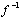.  The domain of f is equal to the range ofand the range of f is the domain of.Example 1:  Use the definition of inverse functions to determine if the functions f and g are inverses of each other: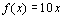and.

 Let’s look at f(g(x)) first: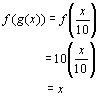*Insert the "value" of g inside the function of f *Plug in the "value" of g wherever there is an x the function f

 Next, let’s look at g(f(x)):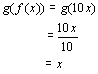*Insert the "value" of f inside the function of g *Plug in the "value" of f wherever there is an x the function g

 Since f(g(x)) AND g(f(x)) BOTH came out to be x, this proves that the two functions are inverses of each other.Example 2:  Use the definition of inverse functions to determine if the functions f and g are inverses of each other: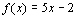and.

 Let’s look at f(g(x)) first:*Insert the "value" of g inside the function of f *Plug in the "value" of g wherever there is an x the function f

 Since BOTH f(g(x)) AND g(f(x)) would have to equal x for them to be inverses of each other and f(g(x)) is not equal to x, then we can stop here and say without a doubt that they are NOT inverses of each other.

 The Horizontal Line Test for Inverse Functions

 If NO horizontal line can be drawn so that it intersects a graph of a function f more than once, then the function f has an inverse functionThe next two examples illustrate this concept.Example 3:  Use the horizontal line test to determine if the graph of the function has an inverse.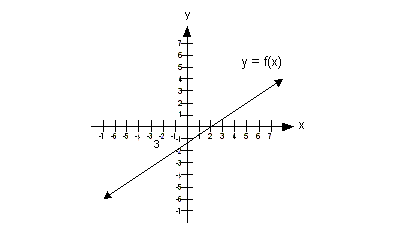This graph would pass the horizontal line test, because there would not be any place on it that we could draw a horizontal line where it would intersect the graph of the function in more than one place.   Therefore, this function has an inverse function.Example 4:  Use the horizontal line test to determine if the graph of the function has an inverse.This function would not pass the horizontal line test because there is at least one place on it that we could draw a horizontal line and intersect it in more than one place.  In fact, there are a lot of horizontal lines that we can draw that would intersect it in more than one place, but we only need to show one to say this function does not have an inverse function. The graph below shows one horizontal line drawn through our graph that intersects it in two places: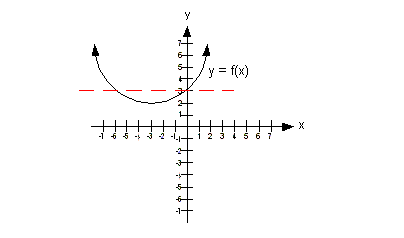Therefore this function does not have an inverse.

 Finding the Inverse of a Function

 Step 1: Replace f(x) with y.

 Step 2: Interchange x with y.

 Step 3: Solve for y.

 If this y is a function, it is the inverse of the original function. If this y is NOT a function, there is no inverse.

 Step 4: If f has an inverse function, then replace y with.Example 5:  Find the equation of the inverse function for the function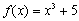.

 Step 1: Replace f(x) with y.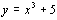Step 2: Interchange x with y.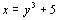Step 3: Solve for y.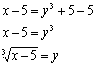*Inverse of - 5 is + 5 *Inverse operation is take the cube root of both side

 Since this y is a function, it is the inverse of the original function.

 Step 4: If f has an inverse function, replace y with.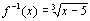Example 6:  Find the equation of the inverse function for the function.

 Step 1: Replace f(x) with y.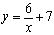Step 2: Interchange x with y.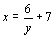Step 3: Solve for y.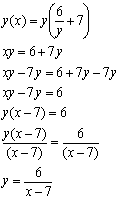*Mult. both sides by the LCD of y *Inverse of -7y is + 7y *Factor out a y *Inverse of mult. by x - 7 is divide by x - 7

 Since this y is a function, it is the inverse of the original function.

 Step 4: If f has an inverse function, replace y with.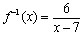Graphing a Function and it's Inverse on the Same Coordinate System

 The graph ofis a reflection of the graph of the function f about the line y = x. The next two examples will illustrate this.Example 7: a) find the equation of,  b) graph f andand c) indicate the domain and range of f andusing interval notation.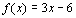a) find the equation ofStep 1: Replace f(x) with y.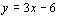Step 2: Interchange x with y.Step 3: Solve for y.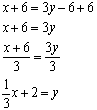*Inverse of -6 is + 6 *Inverse of mult. by 3y is divide by 3y

 Since this y is a function, it is the inverse of the original function.

 Step 4: If f has an inverse function, replace y with.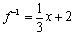b) graph f and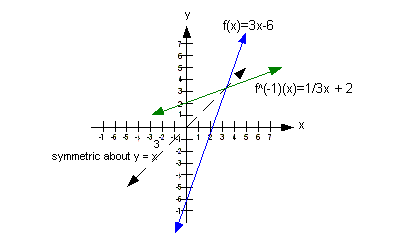See how the graphs of f andare mirrored images across the line y = x?  Note how the ordered pairs are interchanged … for example (2, 0) is a point on f where (0, 2) is a point onIf you need a review on graphing linear functions, feel free to go to College Algebra Tutorial 27:  Graphing Lines.

 c) indicate the domain and range of f andusing interval notation

 The domain of f is the same as the range ofwhich is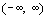. The range of f is the same as the domain ofwhich is. If you need a review on finding the domain and range of a function, feel free to go to College Algebra Tutorial 30: Introduction to Functions. If you need a review on finding the domain and range of a graph of a function, feel free to go to College Algebra Tutorial 32: Graphs of Functions, Part II.Example 8: a) find the equation of,  b) graph f andand c) indicate the domain and range of f andusing interval notation.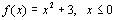a) find the equation ofStep 1: Replace f(x) with y.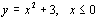Step 2: Interchange x with y.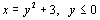Step 3: Solve for y.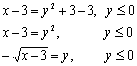*Inverse of +3 is -3 *Inverse of squaring is to take the square root

 Normally when we take the square root of both sides, there are two answers .. the + or – of the square root.  But, because it is only defined for y less than or equal to 0, then that means we can only use the negative square root. If we had both + and – in front of the square root, then this would not be a function and hence there would not be an inverse. But, we do have a function here, so we can carry on …. Since this y is a function, it is the inverse of the original function.

 Step 4: If f has an inverse function, replace y with.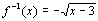b) graph f andSee how the graphs of f andare mirrored images across the line y = x?  Note how the ordered pairs are interchanged … for example (0, 3) is a point on f where (3, 0) is a point on. If you need a review on graphing the quadratic and square root functions, feel free to go to College Algebra Tutorial 31: Graphs of Functions, Part I.

 c) indicate the domain and range of f andusing interval notation

 The domain of f is the same as the range ofwhich is. The range of f is the same as the domain ofwhich is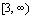. If you need a review on finding the domain and range of a function, feel free to go to College Algebra Tutorial 30: Introduction to Functions. If you need a review on finding the domain and range of a graph of a function, feel free to go to College Algebra Tutorial 32: Graphs of Functions, Part II.Practice Problems

 These are practice problems to help bring you to the next level.  It will allow you to check and see if you have an understanding of these types of problems. Math works just like anything else, if you want to get good at it, then you need to practice it.  Even the best athletes and musicians had help along the way and lots of practice, practice, practice, to get good at their sport or instrument.  In fact there is no such thing as too much practice. To get the most out of these, you should work the problem out on your own and then check your answer by clicking on the link for the answer/discussion for that  problem.  At the link you will find the answer as well as any steps that went into finding that answer.Practice Problems 1a - 1b: Use the definition of inverse functions to determine if the functions f and g are inverses of each other.

 1a.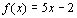and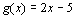(answer/discussion to 1a)

 1b.and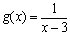(answer/discussion to 1b)Practice Problems 2a - 2b: Given the function: a) find the equation of,  b) graph f andand c) indicate the domain and range of f andusing interval notation.

 2a.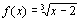(answer/discussion to 2a)

 2b.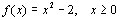(answer/discussion to 2b)Need Extra Help on these Topics?

 There are no appropriate webpages that can assist you in the topics that were covered on this page. Go to Get Help Outside the Classroom found in Tutorial 1: How to Succeed in a Math Class for some more suggestions.

Last revised on June 24, 2010 by Kim Seward.